# Olympiad Test: Fractions and Decimals

## 20 Questions MCQ Test NCERT Mathematics for CAT Preparation | Olympiad Test: Fractions and Decimals

Description
Attempt Olympiad Test: Fractions and Decimals | 20 questions in 20 minutes | Mock test for Class 7 preparation | Free important questions MCQ to study NCERT Mathematics for CAT Preparation for Class 7 Exam | Download free PDF with solutions
QUESTION: 1

### A __________ is a fraction that represents a part of a whole.

Solution:

A proper fraction is a number representing a part of a whole. The whole may be a single object or a group of objects.

Example: Consider the fraction 7/12. This fraction is read as ”seven-twelfth” which means that 7 parts out of 12 equal parts in which the whole is divided.
In the fraction 7/12, 7 is called the numerator and 12 is called the denominator.

QUESTION: 2

### Three times the first of three consecutive odd integers is 3 more than twice the third. The third integer is:

Solution:

Let the three integers be: xx + 2 and x + 4.
Then, 3x = 2(x + 4) + 3

► x = 11.

Third integer = x + 4 = 15.

QUESTION: 3

### The product of two numbers is 2.0016. If one of them is 0.72, find the other number.

Solution:

Product of two numbers = 2.0016
One number = 0.72
Other number = (2.0016 ÷ 0.72)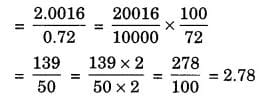Hence, the required number = 2.78

QUESTION: 4

If 2994 ÷ 14.5 = 172, then 29.94 ÷ 1.45 = ?

Solution: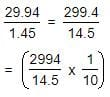[ Here, Substitute 172 in the place of 2994/14.5 ]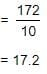QUESTION: 5

The price of commodity X increases by 40 paise every year, while the price of commodity Y increases by 15 paise every year. If in 2001, the price of commodity X was Rs. 4.20 and that of Y was Rs. 6.30, in which year commodity X will cost 40 paise more than the commodity Y ?

Solution:

Suppose commodity X will cost 40 paise more than Y after Z  years. Then,

► (4.20 + 0.40Z) - (6.30 + 0.15Z) = 0.40
► 0.25Z = 0.40 + 2.10
► Z = (2.50 / 0.25) = 10

∴ X will cost 40 paise more than Y 10 years after 2001 i.e in 2011.

QUESTION: 6

A fraction acts as an operator ________.

Solution:

Fraction as an operator ‘of ’.
Fraction acts as an operator of  'of' represents multiplication.

Example: 1/3 of 90 = 1/3 × 90 = 30.

QUESTION: 7

The product of two proper fractions is ________ each of the fractions that are multiplied.

Solution:

When two proper fractions are multiplied, the product is less than both the fractions. Or, we say the value of the product of two proper fractions is smaller than each of the two fractions.

QUESTION: 8

In a class of 40 students 1/5 of the total number of students like to study English, 2/5 of the total number like to study mathematics and the remaining students like to study Science.

Q. How many students like to study English?

Solution:

Number of students who like english = 1/5 of 40

= 1/5 ×40
= 8 students

QUESTION: 9

What will be the vulgar fraction of 0.75

Solution:

► 0.75 = (75 / 100) = 3/4

QUESTION: 10

Find the average of 4.2, 3.8 and 7.6.

Solution:

Average = Sum of numbers / Total quantity of numbers

= (4.2 + 3.8 + 7.6) / 3
= 15.6 / 3
= 5.2

QUESTION: 11

(2 / 7) × 3 =_______

Solution:

► (2 / 7) × 3 = 6 / 7

QUESTION: 12

There is a tree between houses of A and B. If the tree leans on A’s House, the tree top rests on his window which is 12 m from ground. If the tree leans on B’s House, the tree top rests on his window which is 9 m from ground. If the height of the tree is 15 m, what is distance between A’s and B’s house?

Solution: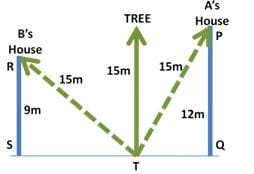In ΔSTR, By Pythagoras theorem:
RT2 = ST2 + RS2

► ST2 = 152 - 92 = 144
► ST = 12m

In ΔTQP, By Pythagoras theorem:
PT2 = TQ2 + PQ2

► TQ2 = 152 - 122 = 81
► TQ = 9m

Distance between houses = SQ = ST + TQ = 12 + 9 = 21m

QUESTION: 13

What decimal of an hour is a second ?

Solution:

Required decimal =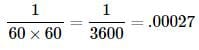QUESTION: 14

(2 / 5) ÷  7 = ______.

Solution:

► (2 / 5) x (1 / 7) = (2 / 35)

QUESTION: 15

0.02 × 0.03 = ______

Solution:

0.02 × 0.03 = 0.0006

QUESTION: 16

In proper fraction, the numerator is ________ the denominator.

Solution:

In a proper fraction, the numerator is always less than the denominator.
Examples of proper fractions include:
1/2, 9/13 and 1/1001.

QUESTION: 17

Reciprocal of 7 / 2 is _____

Solution:

A reciprocal of a fraction a / b is created by changing places of numerator and denominator (it can only be done if the numerator of the original fraction a is different from zero), so it is b / a.

So, in the given example the reciprocal of 7/2 is 2 / 7.

QUESTION: 18

In a class 3/4th of the students do not know either English or Hindi. But 1/6th of the students know English. How much students know both English and Hindi if students who know Hindi are 1/8th of total students in the class?

Solution:

3 / 4th do not know English or Hindi.
So, 1 / 4th know English or Hindi or both.
1/6th know English and 1/8th know Hindi .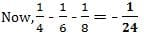QUESTION: 19

(1/3) of 3 is ____

Solution:

(⅓) of 3 means 1/3 * 3 = 1.
∵ 3 in numerator and denominator cancels out.

QUESTION: 20

Multiply 2.05 and 1.3.

Solution:

When you multiply 2.05 by 1.3 you get 2.665 , because there are three decimal places.Use Code STAYHOME200 and get INR 200 additional OFF Use Coupon Code